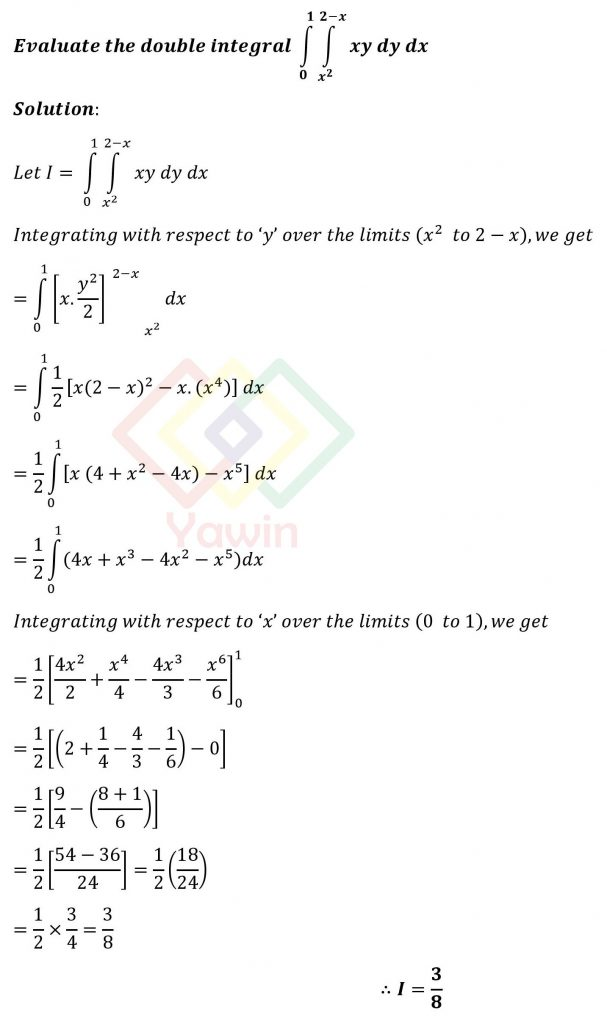Evaluate the double integral of xy dy dx over the limits for y= (x^2 to 2-x) and x=(0 to 1)

## Question## Question

Evaluate the double integral of xy dy dx over the limits for y= (x^2 to 2-x) and x=(0 to 1)